How FEA works, briefly put.

No time to waddle through dozens of pages full of mathematics? Here’s how FEA works – the sticky note version!

1. The governing equation is defined. In magnetostatics, the Ampere’s law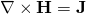is combined with the vector potential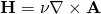to get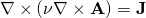.
2. We define an approximative solution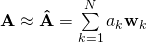.
In other words, known (shape) functions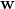multiplied by some unknown coefficients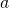.
3. The problem geometry is meshed, and the shape functions are defined based on the mesh.
4. To solve the coefficients, we try to get “as good as possible” solution according to the following criterion: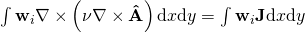for all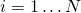.
5. This gives us a system of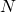equations for ourunknowns.
6. Profit!
7. Lots of awesome figures are drawn (see below).
P.S. To get a unique solution, boundary conditions of course have to be fixed. Typically, the left-hand-side integral is integrated by parts (this also means that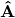doesn’t have to be twice differentiable anymore), after which Neumann boundary conditions (forces, fluxes) can be included in the boundary integral that appears. Dirichlet confitions (fixed displacement, temperature, potential) have to be fixed more manually, by solving only those unknowns that are not directly fixed by this condition.